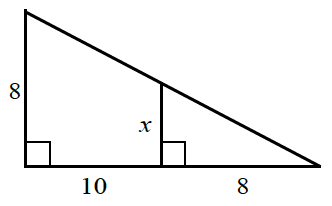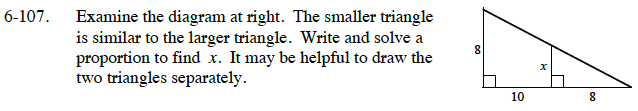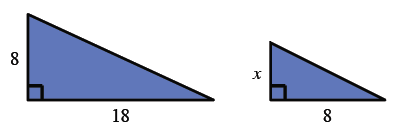### Home > CC3 > Chapter 6 > Lesson 6.2.6 > Problem6-107

6-107.Examine the diagram at right. The smaller triangle is similar to the larger triangle. Write and solve a proportion to find x. It may be helpful to draw the two triangles separately. Homework Help ✎Draw two separate triangles. The larger triangle has base 10 + 8 = 18.$\frac{x}{8}=\frac{8}{18}$